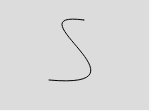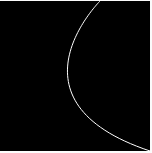# p5.js bezier() function

The bezier() function in p5.js is used to draw cubic Bezier curve on the screen. These curves are defined by a series of anchor and control points.

Syntax:

```bezier( x1, y1, x2, y2, x3, y3, x4, y4 )
or
bezier( x1, y1, z1, x2, y2, z2, x3, y3, z3, x4, y4, z4 )
```

Parameters: The function accepts twelve parameters as mentioned above and described below:

• x1 This parameter stores the x-coordinate for the first anchor point
• y1 This parameter stores the y-coordinate for the first anchor point
• x2 This parameter stores x-coordinate for the first control point
• y2 This parameter stores y-coordinate for the first control point
• x3 This parameter stores x-coordinate for the second control point
• y3 This parameter stores y-coordinate for the second control point
• x4 This parameter stores x-coordinate for the second anchor point
• y4 This parameter stores y-coordinate for the second anchor point
• z1 This parameter stores z-coordinate for the first anchor point
• z2 This parameter stores z-coordinate for the first control point
• z3 This parameter stores z-coordinate for the second control point
• z4 This parameter stores z-coordinate for the second anchor point
• Below programs illustrate the bezier() function in p5.js:

Example 1: This example uses bezier() function to draw a bezier() curve.

 `function` `setup() { ` ` `  `    ``// Create canvas size  ` `    ``createCanvas(150, 110); ` `} ` `  `  `function` `draw() { ` ` `  `    ``// Set the background color ` `    ``background(220); ` `     `  `    ``noFill(); ` `     `  `    ``// Set the stroke color ` `    ``stroke(0, 0, 0); ` `     `  `    ``// Bezier function 8 parameters  ` `    ``// except z-coordinate ` `    ``bezier(85, 20, 10, 10, 160, 90, 50, 80); ` `} `Example 2: This example uses bezier() function with all parameters to draw a bezier() curve.

 `function` `setup() { ` `   `  `    ``// Create canvas size ` `    ``createCanvas(150, 150); ` `} ` ` `  `function` `draw() { ` ` `  `    ``// Set the background color ` `    ``background(0, 0, 0); ` `     `  `    ``noFill(); ` `     `  `    ``// Set the stroke color ` `    ``stroke(255); ` `     `  `    ``// Bezier function with all 12 parameters ` `    ``bezier(150, 150, 0, 100, 100, 0, 100, 0, 0, 0, 100, 0); ` `} `Reference: https://p5js.org/reference/#/p5/bezier

Whether you're preparing for your first job interview or aiming to upskill in this ever-evolving tech landscape, GeeksforGeeks Courses are your key to success. We provide top-quality content at affordable prices, all geared towards accelerating your growth in a time-bound manner. Join the millions we've already empowered, and we're here to do the same for you. Don't miss out - check it out now!

Previous
Next